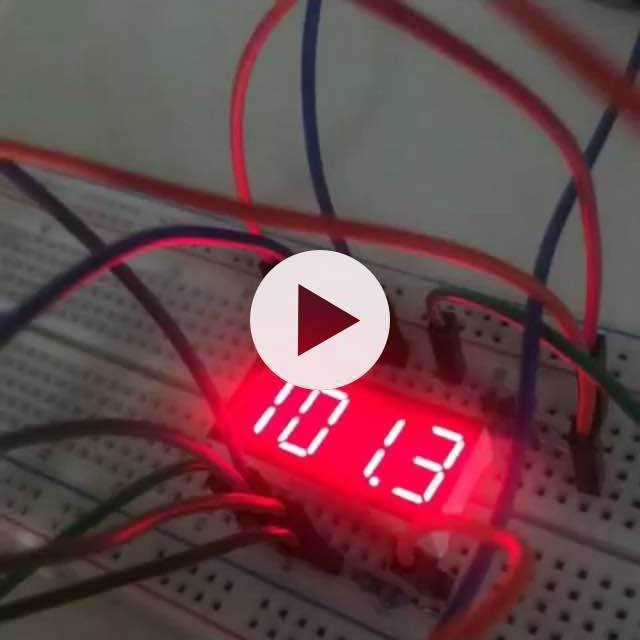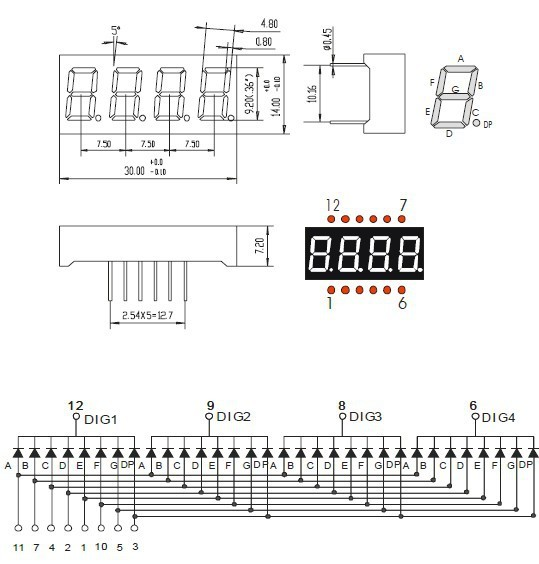08月02, 2016

# 用树莓派驱动八段数码管实现倒计时## 八段数码管## 电压和限流

• Rmax(3.3 - 2) / 0.02 = 130Ω
• Rmin(3.3 - 2) / 0.01 = 65Ω

## 连接电路

• 数码管选择端口：11,13,15,23
• 数码管输出端口：29,31,33,35,37,36,38,40## 实现程序

### 显示数字

``````"use strict";

const Gpio = require("rpio2").Gpio;

const NUMS = [
0b11010111, //0
0b00010001, //1
0b11001011, //2
0b01011011, //3
0b00011101, //4
0b01011110, //5
0b11011110, //6
0b00010011, //7
0b11011111, //8
0b01011111, //9
];

const DP = 0b00100000; //.

var digitGroup = Gpio.group([29,31,33,35,37,36,38,40], true);
digitGroup.open(Gpio.OUTPUT, Gpio.LOW);

digitGroup.value = NUMS; //显示数字 5

//5 秒后换成显示带小数点的数字 3.

setTimeout(function(){
digitGroup.value = NUMS | DP;
}, 5000);

//10 秒后关闭
setTimeout(function(){
digitGroup.close();
}, 10000);
``````

rpio2 版本 V0.4+ 之后支持 GpioGroup，可以通过 `Gpio.group(pins[, activeLow])` 快速创建一组 GPIO 输入。在这里我们创建了负责显示 8 段数字的 group，直接给它输入一个八位二进制数就可以控制显示内容了。

``````const NUMS = [
0b11010111, //0
0b00010001, //1
0b11001011, //2
0b01011011, //3
0b00011101, //4
0b01011110, //5
0b11011110, //6
0b00010011, //7
0b11011111, //8
0b01011111, //9
];

const DP = 0b00100000; //.
``````

``````digitGroup.value = NUMS[n];
``````

``````digitGroup.value = NUMS[n] | DP;
``````

### 切换数码管

``````var portGroup = new Gpio.group([11,13,15,16]);

const wait = require("wait-promise");

//轮流点亮数码管
wait.every(1).and(function(port){
var p = 1 << (port++%4);
digitGroup.value = 0; //将前一个点亮的数码管关闭
portGroup.value = p; //将当前数码管点亮
});
``````

### 两者结合并计时

``````const startTime = Date.now();
const duration = process.argv * 1000;

if(duration <= 0 || duration > 999000){
console.error("Duration must between 1 and 999!");
process.exit(1);
}

wait.every(1).and(function(port){
var time = duration - (Date.now() - startTime);

var p = 1 << (port++%4);
digitGroup.value = 0; //将前一个点亮的数码管关闭
portGroup.value = p;  //将当前数码管点亮

if(time <= 0){
//倒计时结束显示 000.0
digitGroup.value = NUMS | (p & 0b100 ? DP : 0);
return;
}

if(p & 0b1000){
//十分位
digitGroup.value = NUMS[Math.floor(time / 100) % 10];
}else if(p & 0b100){
//个位，要显示一个小数点
digitGroup.value = NUMS[Math.floor(time / 1000) % 10] | DP;
}else if(p & 0b10){
//十位
digitGroup.value = NUMS[Math.floor(time / 10000) % 10];
}else if(p & 0b1){
//百位
digitGroup.value = NUMS[Math.floor(time / 100000) % 10];
}
}).forward();
``````

### 收工

``````"use strict";

const Gpio = require("rpio2").Gpio;
const wait = require("wait-promise");

const NUMS = [
0b11010111, //0
0b00010001, //1
0b11001011, //2
0b01011011, //3
0b00011101, //4
0b01011110, //5
0b11011110, //6
0b00010011, //7
0b11011111, //8
0b01011111, //9
];

const DP = 0b00100000; //.

var digitGroup = Gpio.group([29,31,33,35,37,36,38,40], true);
var portGroup = Gpio.group([11,13,15,16]);

digitGroup.open(Gpio.OUTPUT, Gpio.LOW);
portGroup.open(Gpio.OUTPUT, Gpio.LOW);

const startTime = Date.now();
const duration = process.argv * 1000;

if(duration <= 0 || duration > 999000){
console.error("Duration must between 1 and 999!");
process.exit(1);
}

wait.every(1).and(function(port){
var time = duration - (Date.now() - startTime);

var p = 1 << (port++%4);
digitGroup.value = 0; //将前一个点亮的数码管关闭
portGroup.value = p;  //将当前数码管点亮

if(time <= 0){
//倒计时结束显示 000.0
digitGroup.value = NUMS | (p & 0b100 ? DP : 0);
return;
}

if(p & 0b1000){
//十分位
digitGroup.value = NUMS[Math.floor(time / 100) % 10];
}else if(p & 0b100){
//个位，要显示一个小数点
digitGroup.value = NUMS[Math.floor(time / 1000) % 10] | DP;
}else if(p & 0b10){
//十位
digitGroup.value = NUMS[Math.floor(time / 10000) % 10];
}else if(p & 0b1){
//百位
digitGroup.value = NUMS[Math.floor(time / 100000) % 10];
}
}).forward();

process.on("SIGINT", function(){
digitGroup.close();
portGroup.close();

console.log("shutdown!");
process.exit(0);
});
``````

``````node index.js 100  #倒计时 100 秒
``````

-- EOF --